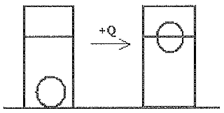# Problem: A uniform density sphere of mass ms, initial radius r0, specific heat c and coefficient of linear expansion α is at rest at the bottom of a bucket of water with density pω. A heater inside the sphere is turned on remotely and generates a heat Q. Since the sphere is well insulated, no heat is transferred to the water; however the heat causes the sphere to expand and rise to the surface. a) What is the buoyant force on the sphere before the heater is turned on? (Give your answer in terms of the given quantities and check units.) b) How much heat, Q, is required to cause the sphere to float to the surface such that half of the sphere is exposed to air? (Give your answer in terms of given quantities and check units.)

###### Problem Details

A uniform density sphere of mass ms, initial radius r0, specific heat c and coefficient of linear expansion α is at rest at the bottom of a bucket of water with density pω. A heater inside the sphere is turned on remotely and generates a heat Q. Since the sphere is well insulated, no heat is transferred to the water; however the heat causes the sphere to expand and rise to the surface.

a) What is the buoyant force on the sphere before the heater is turned on? (Give your answer in terms of the given quantities and check units.)
b) How much heat, Q, is required to cause the sphere to float to the surface such that half of the sphere is exposed to air? (Give your answer in terms of given quantities and check units.)